Home > A2C > Chapter 7 > Lesson 7.2.4 > Problem7-140

7-140.
1. Using your calculator, solve the equations below. Round answers to the nearest 0.001. 7-140 HW eTool (Desmos). Homework Help ✎1. x6 = 125

2. x3.8 = 240

3. x4 = 100

4. (x + 2)3 = 65

5. 4(x − 2)12.5 = 2486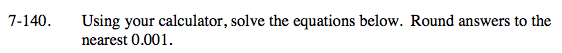Look at problem 7-98.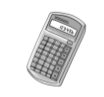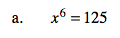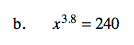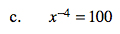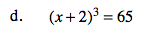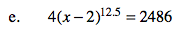Be careful about when and how you remove the exponent. Try to simplify the equation partially first.

2.021

3.673

Use the eTool below to solve the equations.
Click the link at right for the full version of the eTool: A2C 7-140 HW eTool